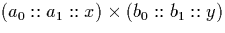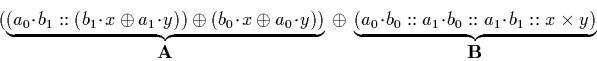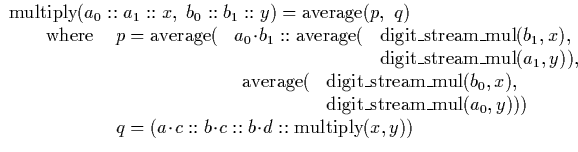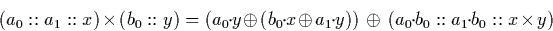Next: Division by an integer Up: Signed Binary Stream Operations Previous: Average

## Multiplication

The algorithm for signed binary stream multiplication is more complex than the dyadic algorithm described in section 4.2.2. We briefly explain why this is so, and show how we can obtain a suitable algorithm. We were unable to find an algorithm for multiplication using this representation in the literature, and so this algorithm is original work.

The algorithm for signed binary stream multiplication is more complex than the dyadic one. This is because the algorithm which averages two signed binary streams requires potentially two digits of each input stream to generate one digit of output, whereas the dyadic stream average algorithm only requires one. The effect of this is that if we attempted to use the same approach for signed binary stream multiplication as for dyadic stream multiplication, we would no longer have an algorithm because the function would be able to recursively call itself indefinitely without ever generating an output digit.

The solution to this problem is to develop an algorithm which uses a lookahead of two on the input streams. Suppose we wish to multiply two streams of the form (a0::a1::x) and (b0::b1::y). We can re-arrange this to show thatis in fact equal to:This may be easily verified by expanding the expressions into their semantic forms using the observations about the `cons' operation and properties of average described in section 3.6.2

We can use this expression to obtain a recursive algorithm. This is because expression A is directly computable using the inputs, three digits of B are available directly without making a recursive call to multiply the streams x and y. We know that average of the streams obtained from terms A and B will require at most (n+1) digits of each to determine n output digits (see sections 4.3.1 and 7.1.2), so in fact two digits may be determined before making a recursive call to the multiplication function.An alternative formulation for which we could also give a recursive algorithm would be:Although superficially simpler, this second algorithm has worse lookahead properties. In fact a better lookahead for signed binary multiplication is achieved by multiplying signed binary digits to dyadics, as described in section 4.2.2, and then converting the resulting dyadic stream back to signed binary (see section 3.7), although we do not use this because it is affected by the problem of swelling dyadic digit representations. These issues are discussed in more detail in chapter 7.Next: Division by an integer Up: Signed Binary Stream Operations Previous: Average
Martin Escardo
5/11/2000